How to read this lecture...

Code should execute sequentially if run in a Jupyter notebook

• See the set up page to install Jupyter, Julia (1.0+) and all necessary libraries
• Please direct feedback to contact@quantecon.org or the discourse forum
• For some notebooks, enable content with "Trust" on the command tab of Jupyter lab
• If using QuantEcon lectures for the first time on a computer, execute ] add InstantiateFromURL inside of a notebook or the REPL

# A First Look at the Kalman Filter¶

## Overview¶

This lecture provides a simple and intuitive introduction to the Kalman filter, for those who either

• have heard of the Kalman filter but don’t know how it works, or
• know the Kalman filter equations, but don’t know where they come from

For additional (more advanced) reading on the Kalman filter, see

The second reference presents a comprehensive treatment of the Kalman filter

Required knowledge: Familiarity with matrix manipulations, multivariate normal distributions, covariance matrices, etc.

### Setup¶

In :
using InstantiateFromURL
github_project("QuantEcon/quantecon-notebooks-julia", version = "0.3.0")

In :
using LinearAlgebra, Statistics


## The Basic Idea¶

The Kalman filter has many applications in economics, but for now let’s pretend that we are rocket scientists

A missile has been launched from country Y and our mission is to track it

Let $x \in \mathbb{R}^2$ denote the current location of the missile—a pair indicating latitude-longitute coordinates on a map

At the present moment in time, the precise location $x$ is unknown, but we do have some beliefs about $x$

One way to summarize our knowledge is a point prediction $\hat x$

• But what if the President wants to know the probability that the missile is currently over the Sea of Japan?
• Then it is better to summarize our initial beliefs with a bivariate probability density $p$

• $\int_E p(x)dx$ indicates the probability that we attach to the missile being in region $E$

The density $p$ is called our prior for the random variable $x$

To keep things tractable in our example, we assume that our prior is Gaussian. In particular, we take

$$p = N(\hat x, \Sigma) \tag{1}$$

where $\hat x$ is the mean of the distribution and $\Sigma$ is a $2 \times 2$ covariance matrix. In our simulations, we will suppose that

$$\hat x = \left( \begin{array}{c} 0.2 \\ -0.2 \end{array} \right), \qquad \Sigma = \left( \begin{array}{cc} 0.4 & 0.3 \\ 0.3 & 0.45 \end{array} \right) \tag{2}$$

This density $p(x)$ is shown below as a contour map, with the center of the red ellipse being equal to $\hat x$

In :
using Plots, Distributions

gr(fmt = :png); # plots setup

In :
# set up prior objects
Σ = [0.4  0.3
0.3  0.45]
x̂ = [0.2, -0.2]

# define G and R from the equation y = Gx + N(0, R)
G = I # this is a generic identity object that conforms to the right dimensions
R = 0.5 .* Σ

# define A and Q
A = [1.2  0
0   -0.2]
Q = 0.3Σ

y = [2.3, -1.9]

# plotting objects
x_grid = range(-1.5, 2.9, length = 100)
y_grid = range(-3.1, 1.7, length = 100)

# generate distribution
dist = MvNormal(x̂, Σ)
two_args_to_pdf(dist) = (x, y) -> pdf(dist, [x, y]) # returns a function to be plotted

# plot
contour(x_grid, y_grid, two_args_to_pdf(dist), fill = false,
color = :lighttest, cbar = false)
contour!(x_grid, y_grid, two_args_to_pdf(dist), fill = false, lw=1,
color = :grays, cbar = false)

Out: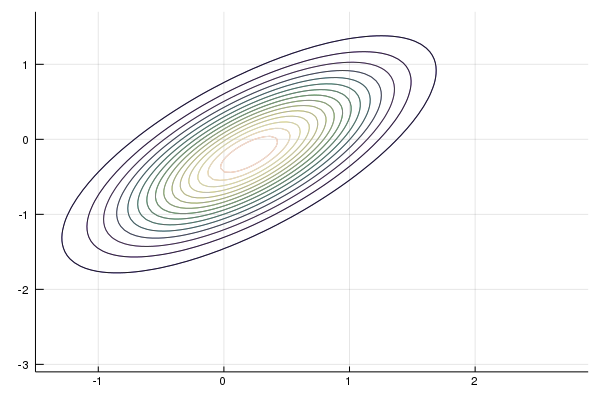### The Filtering Step¶

We are now presented with some good news and some bad news

The good news is that the missile has been located by our sensors, which report that the current location is $y = (2.3, -1.9)$

The next figure shows the original prior $p(x)$ and the new reported location $y$

In :
# plot the figure
annotate!(y, y, "y", color = :black)

Out: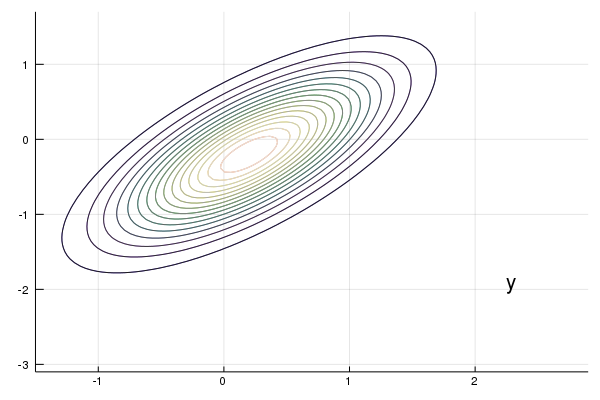The bad news is that our sensors are imprecise.

In particular, we should interpret the output of our sensor not as $y=x$, but rather as

$$y = G x + v, \quad \text{where} \quad v \sim N(0, R) \tag{3}$$

Here $G$ and $R$ are $2 \times 2$ matrices with $R$ positive definite. Both are assumed known, and the noise term $v$ is assumed to be independent of $x$

How then should we combine our prior $p(x) = N(\hat x, \Sigma)$ and this new information $y$ to improve our understanding of the location of the missile?

As you may have guessed, the answer is to use Bayes’ theorem, which tells us to update our prior $p(x)$ to $p(x \,|\, y)$ via

$$p(x \,|\, y) = \frac{p(y \,|\, x) \, p(x)} {p(y)}$$

where $p(y) = \int p(y \,|\, x) \, p(x) dx$

In solving for $p(x \,|\, y)$, we observe that

• $p(x) = N(\hat x, \Sigma)$
• In view of (3), the conditional density $p(y \,|\, x)$ is $N(Gx, R)$
• $p(y)$ does not depend on $x$, and enters into the calculations only as a normalizing constant

Because we are in a linear and Gaussian framework, the updated density can be computed by calculating population linear regressions

In particular, the solution is known  to be

$$p(x \,|\, y) = N(\hat x^F, \Sigma^F)$$

where

$$\hat x^F := \hat x + \Sigma G' (G \Sigma G' + R)^{-1}(y - G \hat x) \quad \text{and} \quad \Sigma^F := \Sigma - \Sigma G' (G \Sigma G' + R)^{-1} G \Sigma \tag{4}$$

Here $\Sigma G' (G \Sigma G' + R)^{-1}$ is the matrix of population regression coefficients of the hidden object $x - \hat x$ on the surprise $y - G \hat x$

This new density $p(x \,|\, y) = N(\hat x^F, \Sigma^F)$ is shown in the next figure via contour lines and the color map

The original density is left in as contour lines for comparison

In :
# define posterior objects
M = Σ * G' * inv(G * Σ * G' + R)
x̂_F = x̂ + M * (y - G * x̂)
Σ_F = Σ - M * G * Σ

# plot the new density on the old plot
newdist = MvNormal(x̂_F, Symmetric(Σ_F)) # because Σ_F
contour!(x_grid, y_grid, two_args_to_pdf(newdist), fill = false,
color = :lighttest, cbar = false)
contour!(x_grid, y_grid, two_args_to_pdf(newdist), fill = false, levels = 7,
color = :grays, cbar = false)
contour!(x_grid, y_grid, two_args_to_pdf(dist), fill = false, levels = 7, lw=1,
color = :grays, cbar = false)

Out: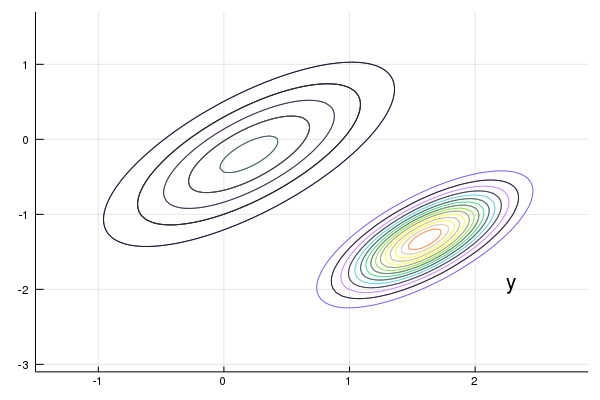Our new density twists the prior $p(x)$ in a direction determined by the new information $y - G \hat x$

In generating the figure, we set $G$ to the identity matrix and $R = 0.5 \Sigma$ for $\Sigma$ defined in (2)

### The Forecast Step¶

What have we achieved so far?

We have obtained probabilities for the current location of the state (missile) given prior and current information

This is called “filtering” rather than forecasting, because we are filtering out noise rather than looking into the future

• $p(x \,|\, y) = N(\hat x^F, \Sigma^F)$ is called the filtering distribution

But now let’s suppose that we are given another task: to predict the location of the missile after one unit of time (whatever that may be) has elapsed

To do this we need a model of how the state evolves

Let’s suppose that we have one, and that it’s linear and Gaussian. In particular,

$$x_{t+1} = A x_t + w_{t+1}, \quad \text{where} \quad w_t \sim N(0, Q) \tag{5}$$

Our aim is to combine this law of motion and our current distribution $p(x \,|\, y) = N(\hat x^F, \Sigma^F)$ to come up with a new predictive distribution for the location in one unit of time

In view of (5), all we have to do is introduce a random vector $x^F \sim N(\hat x^F, \Sigma^F)$ and work out the distribution of $A x^F + w$ where $w$ is independent of $x^F$ and has distribution $N(0, Q)$

Since linear combinations of Gaussians are Gaussian, $A x^F + w$ is Gaussian

Elementary calculations and the expressions in (4) tell us that

$$\mathbb{E} [A x^F + w] = A \mathbb{E} x^F + \mathbb{E} w = A \hat x^F = A \hat x + A \Sigma G' (G \Sigma G' + R)^{-1}(y - G \hat x)$$

and

$$\operatorname{Var} [A x^F + w] = A \operatorname{Var}[x^F] A' + Q = A \Sigma^F A' + Q = A \Sigma A' - A \Sigma G' (G \Sigma G' + R)^{-1} G \Sigma A' + Q$$

The matrix $A \Sigma G' (G \Sigma G' + R)^{-1}$ is often written as $K_{\Sigma}$ and called the Kalman gain

• The subscript $\Sigma$ has been added to remind us that $K_{\Sigma}$ depends on $\Sigma$, but not $y$ or $\hat x$

Using this notation, we can summarize our results as follows

Our updated prediction is the density $N(\hat x_{new}, \Sigma_{new})$ where

\begin{aligned} \hat x_{new} &:= A \hat x + K_{\Sigma} (y - G \hat x) \\ \Sigma_{new} &:= A \Sigma A' - K_{\Sigma} G \Sigma A' + Q \nonumber \end{aligned} \tag{6}

• The density $p_{new}(x) = N(\hat x_{new}, \Sigma_{new})$ is called the predictive distribution

The predictive distribution is the new density shown in the following figure, where the update has used parameters

$$A = \left( \begin{array}{cc} 1.2 & 0.0 \\ 0.0 & -0.2 \end{array} \right), \qquad Q = 0.3 * \Sigma$$
In :
# get the predictive distribution
new_x̂ = A * x̂_F
new_Σ = A * Σ_F * A' + Q
predictdist = MvNormal(new_x̂, Symmetric(new_Σ))

# plot Density 3
contour(x_grid, y_grid, two_args_to_pdf(predictdist), fill = false, lw = 1,
color = :lighttest, cbar = false)
contour!(x_grid, y_grid, two_args_to_pdf(dist),
color = :grays, cbar = false)
contour!(x_grid, y_grid, two_args_to_pdf(newdist), fill = false, levels = 7,
color = :grays, cbar = false)
annotate!(y, y, "y", color = :black)

Out: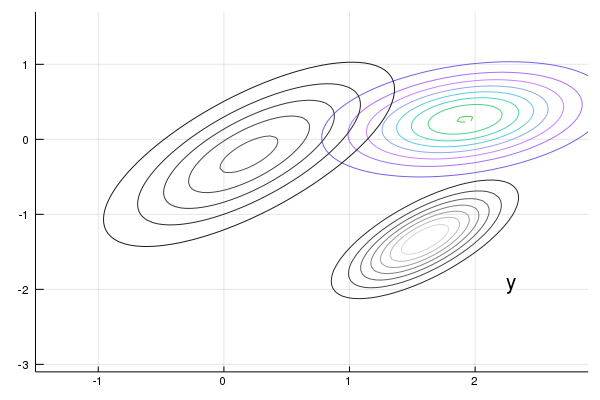### The Recursive Procedure¶

Let’s look back at what we’ve done

We started the current period with a prior $p(x)$ for the location $x$ of the missile

We then used the current measurement $y$ to update to $p(x \,|\, y)$

Finally, we used the law of motion (5) for $\{x_t\}$ to update to $p_{new}(x)$

If we now step into the next period, we are ready to go round again, taking $p_{new}(x)$ as the current prior

Swapping notation $p_t(x)$ for $p(x)$ and $p_{t+1}(x)$ for $p_{new}(x)$, the full recursive procedure is:

1. Start the current period with prior $p_t(x) = N(\hat x_t, \Sigma_t)$
2. Observe current measurement $y_t$
3. Compute the filtering distribution $p_t(x \,|\, y) = N(\hat x_t^F, \Sigma_t^F)$ from $p_t(x)$ and $y_t$, applying Bayes rule and the conditional distribution (3)
4. Compute the predictive distribution $p_{t+1}(x) = N(\hat x_{t+1}, \Sigma_{t+1})$ from the filtering distribution and (5)
5. Increment $t$ by one and go to step 1

Repeating (6), the dynamics for $\hat x_t$ and $\Sigma_t$ are as follows

\begin{aligned} \hat x_{t+1} &= A \hat x_t + K_{\Sigma_t} (y_t - G \hat x_t) \\ \Sigma_{t+1} &= A \Sigma_t A' - K_{\Sigma_t} G \Sigma_t A' + Q \nonumber \end{aligned} \tag{7}

These are the standard dynamic equations for the Kalman filter (see, for example, [LS18], page 58)

## Convergence¶

The matrix $\Sigma_t$ is a measure of the uncertainty of our prediction $\hat x_t$ of $x_t$

Apart from special cases, this uncertainty will never be fully resolved, regardless of how much time elapses

One reason is that our prediction $\hat x_t$ is made based on information available at $t-1$, not $t$

Even if we know the precise value of $x_{t-1}$ (which we don’t), the transition equation (5) implies that $x_t = A x_{t-1} + w_t$

Since the shock $w_t$ is not observable at $t-1$, any time $t-1$ prediction of $x_t$ will incur some error (unless $w_t$ is degenerate)

However, it is certainly possible that $\Sigma_t$ converges to a constant matrix as $t \to \infty$

To study this topic, let’s expand the second equation in (7):

$$\Sigma_{t+1} = A \Sigma_t A' - A \Sigma_t G' (G \Sigma_t G' + R)^{-1} G \Sigma_t A' + Q \tag{8}$$

This is a nonlinear difference equation in $\Sigma_t$

A fixed point of (8) is a constant matrix $\Sigma$ such that

$$\Sigma = A \Sigma A' - A \Sigma G' (G \Sigma G' + R)^{-1} G \Sigma A' + Q \tag{9}$$

Equation (8) is known as a discrete time Riccati difference equation

Equation (9) is known as a discrete time algebraic Riccati equation

Conditions under which a fixed point exists and the sequence $\{\Sigma_t\}$ converges to it are discussed in [AHMS96] and [AM05], chapter 4

A sufficient (but not necessary) condition is that all the eigenvalues $\lambda_i$ of $A$ satisfy $|\lambda_i| < 1$ (cf. e.g., [AM05], p. 77)

(This strong condition assures that the unconditional distribution of $x_t$ converges as $t \rightarrow + \infty$)

In this case, for any initial choice of $\Sigma_0$ that is both nonnegative and symmetric, the sequence $\{\Sigma_t\}$ in (8) converges to a nonnegative symmetric matrix $\Sigma$ that solves (9)

## Implementation¶

The QuantEcon.jl package is able to implement the Kalman filter by using methods for the type Kalman

• Instance data consists of:

• The parameters $A, G, Q, R$ of a given model
• the moments $(\hat x_t, \Sigma_t)$ of the current prior
• The type Kalman from the QuantEcon.jl package has a number of methods, some that we will wait to use until we study more advanced applications in subsequent lectures

• Methods pertinent for this lecture are:

• prior_to_filtered, which updates $(\hat x_t, \Sigma_t)$ to $(\hat x_t^F, \Sigma_t^F)$
• filtered_to_forecast, which updates the filtering distribution to the predictive distribution – which becomes the new prior $(\hat x_{t+1}, \Sigma_{t+1})$
• update, which combines the last two methods
• a stationary_values, which computes the solution to (9) and the corresponding (stationary) Kalman gain

You can view the program on GitHub

## Exercises¶

### Exercise 1¶

Consider the following simple application of the Kalman filter, loosely based on [LS18], section 2.9.2

Suppose that

• all variables are scalars
• the hidden state $\{x_t\}$ is in fact constant, equal to some $\theta \in \mathbb{R}$ unknown to the modeler

State dynamics are therefore given by (5) with $A=1$, $Q=0$ and $x_0 = \theta$

The measurement equation is $y_t = \theta + v_t$ where $v_t$ is $N(0,1)$ and iid

The task of this exercise to simulate the model and, using the code from kalman.jl, plot the first five predictive densities $p_t(x) = N(\hat x_t, \Sigma_t)$

As shown in [LS18], sections 2.9.1–2.9.2, these distributions asymptotically put all mass on the unknown value $\theta$

In the simulation, take $\theta = 10$, $\hat x_0 = 8$ and $\Sigma_0 = 1$

Your figure should – modulo randomness – look something like this### Exercise 2¶

The preceding figure gives some support to the idea that probability mass converges to $\theta$

To get a better idea, choose a small $\epsilon > 0$ and calculate

$$z_t := 1 - \int_{\theta - \epsilon}^{\theta + \epsilon} p_t(x) dx$$

for $t = 0, 1, 2, \ldots, T$

Plot $z_t$ against $T$, setting $\epsilon = 0.1$ and $T = 600$

Your figure should show error erratically declining something like this### Exercise 3¶

As discussed above, if the shock sequence $\{w_t\}$ is not degenerate, then it is not in general possible to predict $x_t$ without error at time $t-1$ (and this would be the case even if we could observe $x_{t-1}$)

Let’s now compare the prediction $\hat x_t$ made by the Kalman filter against a competitor who is allowed to observe $x_{t-1}$

This competitor will use the conditional expectation $\mathbb E[ x_t \,|\, x_{t-1}]$, which in this case is $A x_{t-1}$

The conditional expectation is known to be the optimal prediction method in terms of minimizing mean squared error

(More precisely, the minimizer of $\mathbb E \, \| x_t - g(x_{t-1}) \|^2$ with respect to $g$ is $g^*(x_{t-1}) := \mathbb E[ x_t \,|\, x_{t-1}]$)

Thus we are comparing the Kalman filter against a competitor who has more information (in the sense of being able to observe the latent state) and behaves optimally in terms of minimizing squared error

Our horse race will be assessed in terms of squared error

In particular, your task is to generate a graph plotting observations of both $\| x_t - A x_{t-1} \|^2$ and $\| x_t - \hat x_t \|^2$ against $t$ for $t = 1, \ldots, 50$

For the parameters, set $G = I, R = 0.5 I$ and $Q = 0.3 I$, where $I$ is the $2 \times 2$ identity

Set

$$A = \left( \begin{array}{cc} 0.5 & 0.4 \\ 0.6 & 0.3 \end{array} \right)$$

To initialize the prior density, set

$$\Sigma_0 = \left( \begin{array}{cc} 0.9 & 0.3 \\ 0.3 & 0.9 \end{array} \right)$$

and $\hat x_0 = (8, 8)$

Finally, set $x_0 = (0, 0)$

You should end up with a figure similar to the following (modulo randomness)Observe how, after an initial learning period, the Kalman filter performs quite well, even relative to the competitor who predicts optimally with knowledge of the latent state

### Exercise 4¶

Try varying the coefficient $0.3$ in $Q = 0.3 I$ up and down

Observe how the diagonal values in the stationary solution $\Sigma$ (see (9)) increase and decrease in line with this coefficient

The interpretation is that more randomness in the law of motion for $x_t$ causes more (permanent) uncertainty in prediction

## Solutions¶

In :
using QuantEcon


### Exercise 1¶

In :
# parameters
θ = 10
A, G, Q, R = 1.0, 1.0, 0.0, 1.0
x̂_0, Σ_0 = 8.0, 1.0

# initialize Kalman filter
kalman = Kalman(A, G, Q, R)
set_state!(kalman, x̂_0, Σ_0)

xgrid = range(θ - 5, θ + 2, length = 200)
densities = zeros(200, 5) # one column per round of updating
for i in 1:5
# record the current predicted mean and variance, and plot their densities
m, v = kalman.cur_x_hat, kalman.cur_sigma
densities[:, i] = pdf.(Normal(m, sqrt(v)), xgrid)

# generate the noisy signal
y = θ + randn()

# update the Kalman filter
update!(kalman, y)
end

labels = ["t=1", "t=2", "t=3", "t=4", "t=5"]
plot(xgrid, densities, label = labels, legend = :topleft, grid = false,
title = "First 5 densities when theta = $θ")  Out: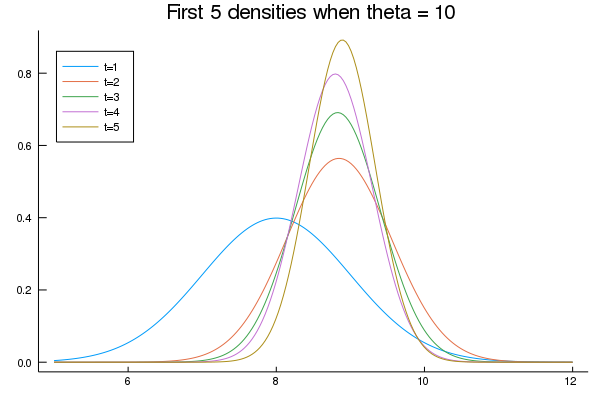### Exercise 2¶ In : using Random, Expectations Random.seed!(43) # reproducible results ϵ = 0.1 kalman = Kalman(A, G, Q, R) set_state!(kalman, x̂_0, Σ_0) nodes, weights = qnwlege(21, θ-ϵ, θ+ϵ) T = 600 z = zeros(T) for t in 1:T # record the current predicted mean and variance, and plot their densities m, v = kalman.cur_x_hat, kalman.cur_sigma dist = Truncated(Normal(m, sqrt(v)), θ-30*ϵ, θ+30*ϵ) # define on compact interval, so we can use gridded expectation E = expectation(dist, nodes) # nodes ∈ [θ-ϵ, θ+ϵ] integral = E(x -> 1) # just take the pdf integral z[t] = 1. - integral # generate the noisy signal and update the Kalman filter update!(kalman, θ + randn()) end plot(1:T, z, fillrange = 0, color = :blue, fillalpha = 0.2, grid = false, xlims=(0, T), legend = false)  Out: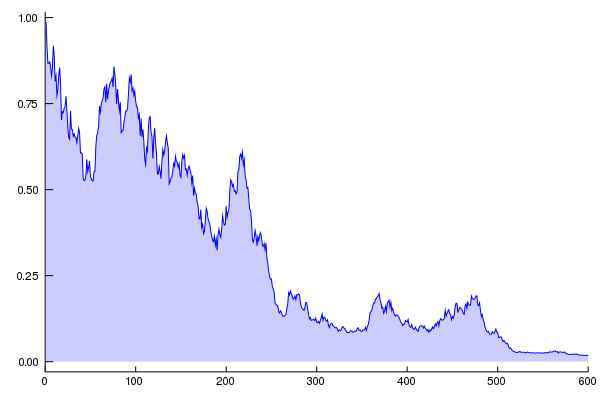### Exercise 3¶ In : # define A, Q, G, R G = I + zeros(2, 2) R = 0.5 .* G A = [0.5 0.4 0.6 0.3] Q = 0.3 .* G # define the prior density Σ = [0.9 0.3 0.3 0.9] x̂ = [8, 8] # initialize the Kalman filter kn = Kalman(A, G, Q, R) set_state!(kn, x̂, Σ) # set the true initial value of the state x = zeros(2) # print eigenvalues of A println("Eigenvalues of A:\n$(eigvals(A))")

# print stationary Σ
S, K = stationary_values(kn)
println("Stationary prediction error variance:\n\$S")

# generate the plot
T = 50
e1 = zeros(T)
e2 = similar(e1)
for t in 1:T

# generate signal and update prediction
dist = MultivariateNormal(G * x, R)
y = rand(dist)
update!(kn, y)

# update state and record error
Ax = A * x
x = rand(MultivariateNormal(Ax, Q))
e1[t] = sum((a - b)^2 for (a, b) in zip(x, kn.cur_x_hat))
e2[t] = sum((a - b)^2 for (a, b) in zip(x, Ax))
end

plot(1:T, e1, color = :black, linewidth = 2, alpha = 0.6, label = "Kalman filter error",
grid = false)
plot!(1:T, e2, color = :green, linewidth = 2, alpha = 0.6,
label = "conditional expectation error")

Eigenvalues of A:
[-0.10000000000000003, 0.8999999999999999]
Stationary prediction error variance:
[0.4032910794778669 0.10507180275061759; 0.1050718027506176 0.41061709375220456]

Out: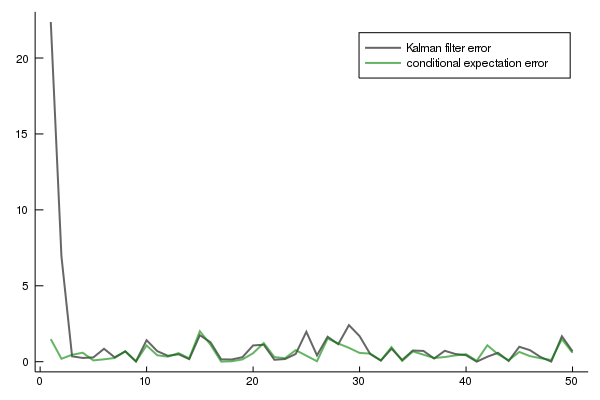Footnotes

 See, for example, page 93 of [Bis06]. To get from his expressions to the ones used above, you will also need to apply the Woodbury matrix identity.

• Share page